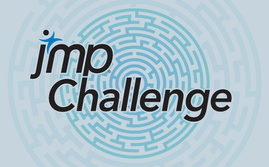Choose Language Hide Translation Bar
Challenge 3In last month’s challenge, I had to avoid creating a situation where overflow error might cause additional complications. In fact, the algorithm for modular exponentiation in the Miller-Rabin based isPrime routine is written to avoid this problem. Inevitably, you will run across this issue regardless of the programming language.

An overflow error occurs when you try to perform an operation requiring more bits than are available to represent the results. This often, but not exclusively, occurs when working with large integers. For example, try the following examples:

``````//Example 1
x = 9007199254740992;
For(i=1,i<=10,i++,Print(x+i));
x = 9007199254740992;
For(i=1,i<=10,i++,Print(x-i));

//Example 2
n1 = 9007199254740999;
n2 = 1;
Print(n1-n2);``````

253 is the largest integer that JMP can represent before overflow happens. Here’s a nice explanation of floating-point representation (all numbers in JMP are floating point). For this month’s challenge, I would like to see you come up with methods for adding and subtracting numbers that would overflow JMP’s representation of integers. Create two functions

1. subtractBig(n1,n2) where n1 and n2 are both integers (positive, negative, or 0) in text form. Returns a value in text form.

n1 – integer in text form or, if n2 is empty, a list of integers (positive, negative, or 0) in text form.

n2 – integer in text form

Returns a value in text form.

Examples

subtractBig(“9007199254740999”, “9007199254740998”) returns “1”

Scoring

• 5 points for a version of addBig that can 1) add two numbers of at most 1000 digits in under 30 seconds, and 2) find the sum of a list containing at most 100, 100-digit numbers in under 30 seconds. Part 1) will be tested with 10 different sets of numbers. The number of digits in each number will not necessarily be the same. The same set of 10 will be used for all submissions.
• 3 additional points for subtractBig, which must compute (x – y), where x and y are numbers of at most 1000 digits, in under 30 seconds. Ten different sets of numbers will be used. The number of digits for each number will not necessarily be the same. The same set of 10 will be used for all submissions.
• 3 bonus points for the addBig submission that can correctly add the largest (number of digit) n1 and n2 of equal lengths, in under 5 seconds. (2 points for 2nd, 1 for the 3rd)
• 3 bonus points for the addBig submission that can correctly add the most 1000-digit numbers in under 5 seconds (2 points for 2nd, 1 point for 3rd).
• 3 extra points each if any submission is as fast or faster than any submission by a JMP staff member.

Submission deadline is 11:59 PM Eastern (US) time Monday, June 15. Good luck!

Article Labels
Article TagsSuper User

For the case where we are adding the list of numbers, can we assume that each number is the same length or is this something that we need to test for? (i.e. do we need to handle the general case or just the test scenario that you have described?)Super User

In response to my query - I've just read your 3rd example - this would suggest (i)  that we can't assume that all the numbers in the n1 list have the same number of digits.  Also, (ii) it could be that the n1 list contains negative numbers.  Presumably then (iii) for the case of two  numbers n1 and n2 one or both of those could be negative?

Perhaps you can just clarify the 3 points I've mentioned. Thanks.Super User

Don

I read your challenge description again last night, and I think you give a clear explanation of the requirements.  Feel free to disregard my queries.  Sorry about that!

-DaveStaff

It's probably worth clarifying, since this is a bit confusing. Both functions (and both cases for addBig) should be able to handle numbers of different lengths and signs. I will test this. When determining bonus speed and size points, I will use numbers of the same length.Staff

Just a reminder. Tonight is the deadline for Challenge 3 submissions.